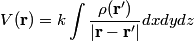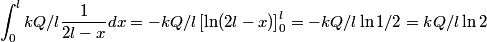## Solution to 1986 Problem 98

 The potential due to a localized charge distribution is given by\begin{align*}V(\mathbf{r}) = k \int \frac{\rho(\mathbf{r'})}{|\mathbf{r} - \mathbf{r'}|} dx dy dz\end{align*}So, the potential due to glass rod at point P is\begin{align*}\int_0^l k Q/l \frac{1}{2l - x} dx = -k Q/ l \left [ \ln (2l- x)\right]_0^l = -k Q/ l \ln 1/2 = kQ/l \ln 2\end{...Therefore, answer (D) is correct.